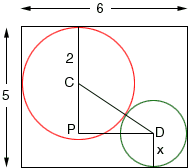Question: Given a rectangle with dimensions L=6, H=5. Two circles are inscribed such that they touch each other(circles are adjacent to each other) and also their circumferences touch 2 sides of the rectangle. One of the circles has radius=4. Find the radius of the other circle. I would really appreciate if you could tell me how to arrive at the answer. Thank you Amina Hi Amina, I think that the circle must have a diameter of 4 units, otherwise it would not fit inside the rectangle. I drew a diagram below with one circle having radius 2 units (diameter 4 units) and the other having an unknown radius of x units.The right triangle CPD has |CP| = 5 - 2 - x, |PD| = 6 - 2 - x and |DC| = x + 2 The theorem of Pythagoras implies that |CP|2 + |PD|2 = |DC|2 This gives a quadratic in x which you can solve. Cheers, Penny Go to Math Central